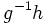# Cayley graph of a group

(diff) ← Older revision | Latest revision (diff) | Newer revision → (diff)
Let$G$ be a group and$S$ be a generating set for$G$. The Cayley graph of$G$ with respect to$S$ is defined as follows:
• The vertex set of the graph is$G$.
• Given two distinct vertices$g,h \in G$, there is an edge joining$g$ to$h$ if and only if$g^{-1}h$ is in$S \cup S^{-1}$.
We typically consider the Cayley graph for a finitely generated group and a finite generating set of the group. Further, we can assume without loss of generality that$S$ is a symmetric subset of$G$ -- the inverse of any element of$S$ is also in$S$.